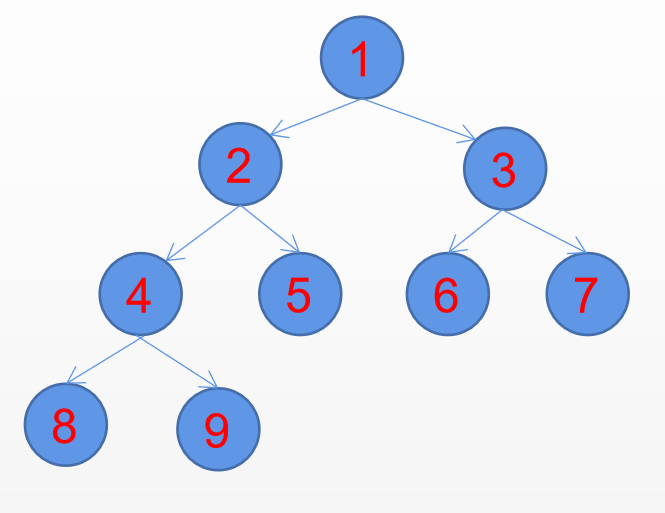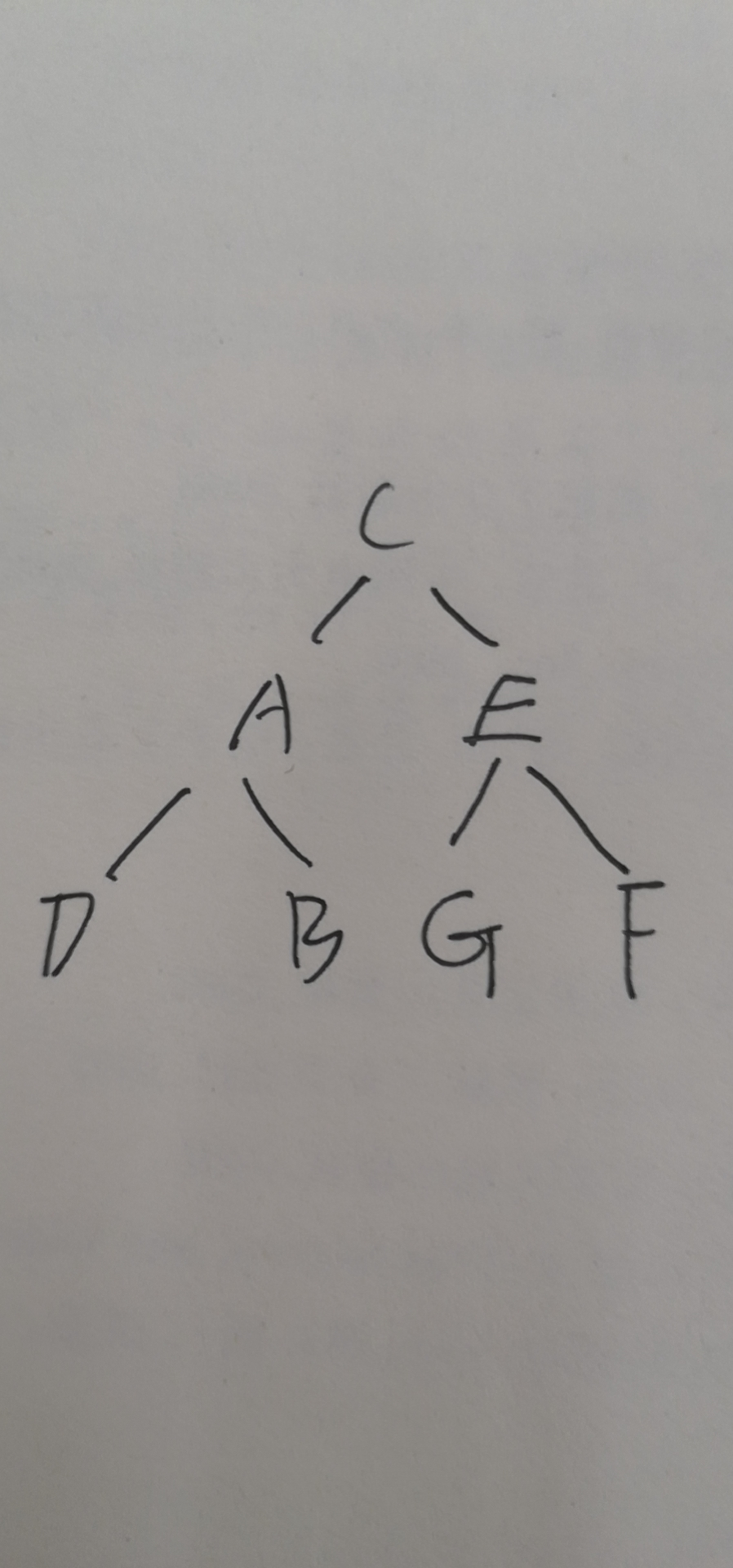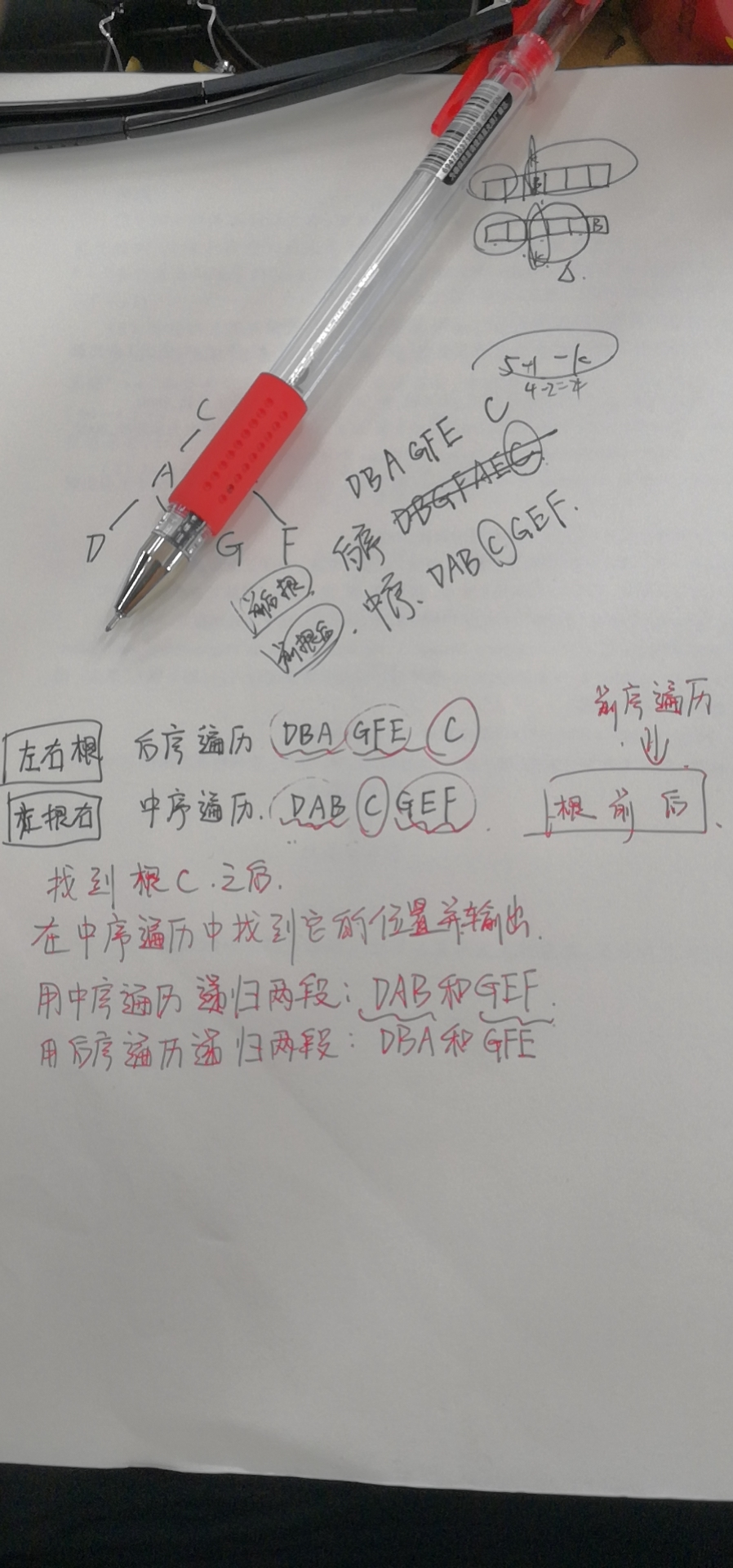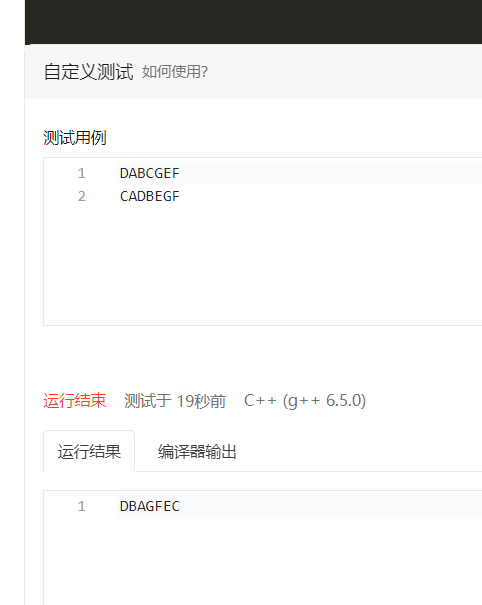• 后序遍历 + 中序遍历 --------------- 可以确定唯一一颗二叉树 前序遍历 + 中序遍历 --------------- 不能唯一确定 ！因为先序后序遍历都是确定根的位置，但不能确定左右子树的位置，一颗二叉树的建立需要根的位置也...
二叉树的遍历我们知道知道 前序遍历 + 中序遍历 --------------- 可以确定唯一一棵二叉树 后序遍历 + 中序遍历 --------------- 可以确定唯一一颗二叉树 前序遍历 + 中序遍历 --------------- 不能唯一确定
！因为先序后序遍历都是确定根的位置，但不能确定左右子树的位置，一颗二叉树的建立需要根的位置也需要左右子树的位置。
根据前序遍历和中序遍历重建二叉树
首先来看下这两种遍历的方式， 前序遍历的遍历是：根节点 – 左子树 – 右子树 , 中序遍历是 左子树 – 根节点 – 右子树. 故前序遍历的第一个节点必然是二叉树的根节点， 来看上面二叉树的前序遍历 : a - b - d - e - c - f - g; 故可以确定a就是二叉树的根节点。 然后我们在中序遍历中找到a这个节点。 d - b - e - (a) - f - c - g; 根据中序遍历先遍历左子树，再遍历根节点最后遍历右子树的这个特点，我们就知道了中序遍历中a的左边 d b e 是a的左子树部分， 因为中序遍历必定先遍历完根节点的左子树部分再遍历根节点， 故a的右边部分 f c g 就是a的右子树部分。 故接下来的过程就是找到a的左子树节点和a的右子树节点， 故a的左子树节点必然在中序遍历的 a 左边的部分， a 的右子树节点必定在中序遍历的a的右半部分, 以找a左子树节点为例子，此时我们知道了其左子树就是 d - b - e， 这三个节点， 而再看前序遍历， 前序遍历要先遍历根节点再遍历左子树， 故前序遍历中a后面的三个节点即为左子树的三个节点，其第一个节点即为左子树根节点。故b为a的左子树节点，同样我们以b为根节点的子树在中序遍历找到b的位置， 其位置为 d - （b） - e - a - f - c - g; 其左边为只有一个d为其左子树，右边只有一个e为其右子树。 同样找a右子树的时候， 由中序遍历知道了 f - c - g 是 a 的右子树， 故在前序遍历中知道了第一个是根节点a, 又知道其先遍历了3个左子树节点，故第五个节点开始遍历右子树，其第五个节点就是右子树的根节点。 以此递归，看下面的代码实现
/*
这里并没有专门写一个类或者结构体，表示一个节点(包括存放的数据和左右子树的指针)
直接用map表示每一个节点的左子树是那个节点和右子树是那个节点.
*/
#include<iostream>
#include<queue>
#include<unordered_map>
using namespace std;
constexpr int N = 40;
int pre[N], in[N];
unordered_map<int, int> l, r, pos;

int Recstr_Tree(int il, int ir, int pl, int pr)
{
int root = pre[pl];            // 可知前序遍历最先遍历根节点
int k = pos[root];             // pos用 map快速找到根节点在中序遍历的位置

// 若il < k，即中序遍历的左边界小于当前根节点在中序遍历的节点位置，说明该根节点存在左子树, 进行递归
// 故此时递归时, 左边子树的中序范围为 il ~ k - 1, 前序遍历的左边界要向前移动故 pl + 1, 然后前序的
// 右边界如何确定， 可得 (k - 1) - il = x - (pl + 1)  -->  x = pl + k - il;
if(il < k) l[root] = Recstr_Tree(il, k - 1, pl + 1, pl + k - il);

if(ir > k) r[root] = Recstr_Tree(k + 1, ir, pl + k - il + 1, pr);

return root;
}

// 层序遍历
auto level_traversal(int root)
{
queue<int> q;
q.push(root);
while(q.size())
{
int node = q.front(); q.pop();
cout << node << " ";
if(l[node]) q.push(l[node]);
if(r[node]) q.push(r[node]);
}
}

auto main() -> int
{
int n; cin >> n;
for(int i = 0; i < n; ++i) cin >> pre[i];    // 输入前序遍历
for(int i = 0; i < n; ++i)
{
cin >> in[i];                           // 输入中序遍历
pos[in[i]] = i;                         // 保存每个节点在中序遍历的位置
}
int root = Recstr_Tree(0, n - 1, 0, n - 1); // 重建树
level_traversal(root);                      // 进行层序遍历
cout << endl;
return 0;
}

根据后序遍历和中序遍历重建二叉树
相关题目的链接 ： AcWing 1497. 树的遍历
同样的话根据后序遍历 和 中序遍历重建二叉树也是同理，后序遍历是先遍历左子树 – 遍历右子树 – 最后遍历根节点， 故每次确定根节点应该是用后序遍历的右边界来确定， 故代码实现基本差不多 .
#include<iostream>
#include<queue>
#include<unordered_map>
using namespace std;
constexpr int N = 40;
int post[N], in[N];
unordered_map<int, int> l, r, pos;

int Recstr_Tree(int il, int ir, int pl, int pr)
{
int root = post[pr];
int k = pos[root];
if(il < k) l[root] = Recstr_Tree(il, k - 1, pl, pl + k - il - 1);
if(ir > k) r[root] = Recstr_Tree(k + 1, ir, pl + k - il, pr - 1);
return root;
}

auto level_traversal(int root)
{
queue<int> q;
q.push(root);
while(q.size())
{
int node = q.front(); q.pop();
cout << node << " ";
if(l[node]) q.push(l[node]);
if(r[node]) q.push(r[node]);
}
}

auto main() -> int
{
int n; cin >> n;
for(int i = 0; i < n; ++i) cin >> post[i];
for(int i = 0; i < n; ++i)
{
cin >> in[i];
pos[in[i]] = i;
}
int root = Recstr_Tree(0, n-1, 0, n-1);
level_traversal(root);
cout << endl;
return 0;
}

展开全文数据结构 算法 c++
• 二叉树的遍历规则主要有三种：前序遍历，中序遍历后序遍历。它们是根据访问根节点的先后顺序来划分的。 前序遍历： 1.访问根节点 2.前序遍历左子树 3.右序遍历右子树 中序遍历： 1.中序遍历左子树 2.访问根节点...
二叉树的遍历规则主要有三种：前序遍历，中序遍历，后序遍历。它们是根据访问根节点的先后顺序来划分的。
前序遍历：

1.访问根节点 2.前序遍历左子树 3.右序遍历右子树

中序遍历：

1.中序遍历左子树 2.访问根节点 3.中序遍历右子树

后序遍历：

1.后序遍历左子树 2.后序遍历右子树 3.访问根节点如上图
前序遍历：1 2 4 8 9 5 3 6 7 中序遍历：8 4 9 2 5 1 6 3 7 后序遍历：8 9 4 5 2 6 7 3 1 层次遍历：1 2 3 4 5 6 7 8 9
class Node():
# 节点类
def __init__(self, data=-1):
self.data = data
self.left = None
self.right = None

class Tree():
# 树类
def __init__(self):
self.root = Node()

# 为树加入节点
node = Node(data)
if self.root.data == -1:  # 如果树为空，就对根节点赋值
self.root = node
else:
myQueue = [self.root]
while myQueue:  # 对已有的节点进行层次遍历
treeNode = myQueue.pop(0)
if not treeNode.left:
treeNode.left = node
return
elif not treeNode.right:
treeNode.right = node
return
else:
myQueue.append(treeNode.left)
myQueue.append(treeNode.right)

def pre_order_recursion(self, root):  # 递归实现前序遍历
if not root:
return
print(root.data)
self.pre_order_recursion(root.left)
self.pre_order_recursion(root.right)

def in_order_recursion(self, root):  # 递归实现中序遍历
if not root:
return
self.in_order_recursion(root.left)
print(root.data)
self.in_order_recursion(root.right)

def post_order_recursion(self, root):  # 递归实现后序遍历
if not root:
return
self.post_order_recursion(root.left)
self.post_order_recursion(root.right)
print(root.data)

def pre_order_stack(self, root):  # 堆栈实现前序遍历（非递归）
if not root:
return
myStack = []
node = root
while myStack or node:
while node:  # 从根节点开始，一直寻找他的左子树
print(node.data)
myStack.append(node)
node = node.left
node = myStack.pop()  # while结束表示当前节点node为空，即前一个节点没有左子树了
node = node.right  # 开始查看它的右子树

def in_order_stack(self, root):  # 堆栈实现中序遍历（非递归）
if not root:
return
myStack = []
node = root
while myStack or node:  # 从根节点开始，一直寻找它的左子树
while node:
myStack.append(node)
node = node.left
node = myStack.pop()  # while结束表示当前节点node为空，即前一个节点没有左子树了
print(node.data)

node = node.right  # 开始查看它的右子树

def post_order_stack(self, root):  # 堆栈实现后序遍历（非递归）
# 先遍历根节点，再遍历右子树，最后是左子树，这样就可以转化为和先序遍历一个类型了，最后只把遍历结果逆序输出就OK了。
if not root:
return
myStack1 = []
myStack2 = []
node = root
while myStack1 or node:
while node:
myStack2.append(node)
myStack1.append(node)
node = node.right
node = myStack1.pop()
node = node.left
while myStack2:
print(myStack2.pop().data)

def level_order_queue(self, root):  # 队列实现层次遍历（非递归）
if not root:
return
myQueue = []
node = root
myQueue.append(node)
while myQueue:
node = myQueue.pop(0)
print(node.data)
if node.left:
myQueue.append(node.left)
if node.right:
myQueue.append(node.right)

if __name__ == '__main__':
# 主函数
datas = [1, 2, 3, 4, 5, 6, 7, 8, 9]
tree = Tree()  # 新建一个树对象
for data in datas:
#
print('递归实现前序遍历：')
tree.pre_order_recursion(tree.root)

print('\n堆栈实现前序遍历')
tree.pre_order_stack(tree.root)

print("\n\n递归实现中序遍历：")
tree.in_order_recursion(tree.root)

print("\n堆栈实现中序遍历：")
tree.in_order_stack(tree.root)

print('\n\n递归实现后序遍历：')
tree.post_order_recursion(tree.root)

print('\n堆栈实现后序遍历：')
tree.post_order_stack(tree.root)

print('\n\n队列实现层次遍历：')
tree.level_order_queue(tree.root)


展开全文数据结构
• 给树的后序和中序遍历，求先序遍历 假设有一棵树长这样 很容易写出他的后序遍历DBAGFEC 也很容易写出他中序遍历DABCGEF 1.后序遍历是前后根，根节点永远是最后一个，因此我们可以找到根节点c 2.要我们输出前序遍历...给树的后序和中序遍历，求先序遍历 假设有一棵树长这样很容易写出他的后序遍历DBAGFEC 也很容易写出他中序遍历DABCGEF1.后序遍历是前后根，根节点永远是最后一个，因此我们可以找到根节点c 2.要我们输出前序遍历，因为根节点永远在前面，所以每次找到就输出就好了 3.之后用find函数找到根节点c在中序遍历中的位置，划分为两段DAB GEF，然后中序遍历这两段，注意一定要是先递归DAB再递归GEF不然的话就是根后前而不是根前后了 4.也将后序遍历划分为两段DAB GFE，用后序遍历递归下去
好了，直接上代码
#include<iostream>
#include<algorithm>
#include<cstring>
using namespace std;
void dg(string a,string b) {
if (a.size() > 0) {
char c = b[b.size() - 1];
cout << c;
int k = a.find(c);
dg(a.substr(0, k), b.substr(0, k));
dg(a.substr(k + 1), b.substr(k ,b.size()-k-1));
}·
}
int main() {
ios::sync_with_stdio(false);
string in,aft;
cin >> in >> aft;
dg(in, aft);
}

给出前序遍历和中序遍历求后序遍历
#include<iostream>

using namespace std;
void dfs(string a, string b) {
if (a.size() <= 0)return;
char c = b;

int x = a.find(c);
dfs(a.substr(0, x), b.substr(1, x));
dfs(a.substr(x + 1), b.substr(b.size()-x));
cout << c;
}
int main() {
string a, b;
cin >> a >> b;
dfs(a, b);
}给出前序遍历和后序遍历求中序遍历


展开全文• 输入某二叉树后序遍历中序遍历的结果，请输出前序遍历序列。假设输入的后序遍历中序遍历的结果中都不含重复的数字。例如输入后序遍历序列{7,1,4,2,5,8,6,3,1}和中序遍历序列{4,7,2,1,5,3,8,6}，重建二叉树并...
描述

输入某二叉树的后序遍历和中序遍历的结果，请输出前序遍历序列。假设输入的后序遍历和中序遍历的结果中都不含重复的数字。例如输入后序遍历序列{7,1,4,2,5,8,6,3,1}和中序遍历序列{4,7,2,1,5,3,8,6}，重建二叉树并返回后序遍历序列

输入

输入某二叉树的后序遍历和中序遍历的结果

输出

输出前序遍历序列

输入样例 1

7 4 2 5 8 6 3 1 4 7 2 1 5 3 8 6

输出样例 1

1 2 4 7 3 5 6 8

参考题目详解：已知前序遍历和中序遍历求二叉树
实现代码：
#include<stdio.h>
#include<stdlib.h>
#include<string.h>
typedef struct node* BinTree;
struct node{
int date;
BinTree left;
BinTree right;
};
BinTree Build(int post[] ,int in[],int size)
{
if(size<=0)return NULL;
//先在中序中找到根节点
int i=0;
for(i=0;i<size;i++)
{
if(in[i]==post[size-1])break;
}
BinTree tree=(BinTree)malloc(sizeof(struct node));
tree->date=post[size-1];
tree->left=Build(post,in,i);
tree->right=Build(post+i,in+i+1,size-1-i);
return tree;
}

void preorder(BinTree T)  //前序遍历
{
if(T==NULL)return;
else{
printf("%d ",T->date);
preorder(T->left);
preorder(T->right);
}

}
int main()
{
char postt,inn;
gets(postt);
gets(inn);
int size=strlen(postt);
int post,in;
int postcount=0;
int i;
for(i=0;i<size;i++)
{
if(i==0)
{
post[postcount]=postt[i]-'0';
}
else
{
if(postt[i]>='0'&&postt[i]<='9')
{
//此if-else 用来转换多位数为int类型
if(postt[i-1]==' ')  //如果前一个元素为空格
{
postcount++;
post[postcount]=postt[i]-'0';
}

else  //如果前一个元素不是空格，那么说明与前一个元素一同构成的数  例如：10
{
post[postcount]=post[postcount]*10+(postt[i]-'0');
}
}

}

}
int incount=0;
for(i=0;i<size;i++)
{
if(i==0)
{
in[incount]=inn[i]-'0';
}
else
{
if(inn[i]>='0'&&inn[i]<='9')
{
if(inn[i-1]==' ')
{
incount++;
in[incount]=inn[i]-'0';
}

else
{
in[incount]=in[incount]*10+(inn[i]-'0');
}
}

}

}
//如果后序遍历的结点数与中序遍历的结点数相同且不为0，那么可以找到对应二叉树
if(postcount==incount&&postcount!=0)
{
BinTree T;
T=Build(post,in,postcount+1);
preorder(T);
}

return 0;

}

展开全文前序遍历
• 根据二叉树后序遍历以及中序遍历还原二叉树 【题目】假设一棵二叉树后序遍历序列为 DGJHEBIFCA ，中序遍历序列为 DBGEHJACIF ，则其前序遍历序列为 ( ) 。A. ABCDEFGHIJB. ABDEGHJCFIC. ABDEGHJFICD. ...
• 二叉树的前序遍历 中序遍历 后序遍历 层次遍历的递归与非递归实现算法
• 题目描述 二叉树的前序、中序、后序遍历的定义： 前序遍历：对任一子树，先访问跟，然后遍历 其左子树，最后遍历其右子树； 中序遍历：对任一子树，...前序遍历与中序遍历能够唯一确定后序遍历）。 输入 两个
• 先序遍历和中序遍历确定二叉树 后序遍历中序遍历确定二叉树递归
• 1.已知先序遍历、中序遍历后序遍历中的任意一种方式，都无法建立起二叉树。 2.已知先序遍历和中序遍历，可以建立二叉树。 3.已知中序遍历后序遍历，可以建立二叉树。 4.已知先序遍历和后序遍历，无法建立...
• 已知前序遍历中序遍历，求二叉树后序遍历前序遍历与中序遍历规律与方法分析步骤代码实现 前序遍历与中序遍历 数组下标 0 1 2 3 4 5 6 7 前序遍历 7 4 1 6 5 3 8 2 中序遍历 1 4 5 6 7 8 3 2 规律与...
• 中序遍历后序遍历重建二叉树 中序遍历中，根节点总是位于左右子树中间，将左右子树分开。 后序遍历中，根节点总是在左右子树之后。 重建算法： 现在说一下重建根节点的过程，其他节点可以递归建立。 由后序遍历...ACM python
• 二叉树1 前序遍历2 中序遍历3 后序遍历4 层次遍历5 二叉树的查找6 二叉树的插入7 二叉树的删除 二叉树(有序)查找既可以兼顾查找速度有可以兼顾查找后的插入与删除的实现(减少时间和空间的冗余)。 话不多说，先把各种...python 数据结构 队列 算法
• 中序遍历：先遍历二叉树的左孩子再遍历根再遍历右孩子。 后序遍历：先遍历二叉树的左孩子再遍历右孩子再遍历根。 从种遍历方式上可以分析出的规律有 a、前序遍历的根一定是第一个。 b、后序遍历的根一定是最后一...
• 前序遍历和后序遍历确定的是根的位置，而中序遍历二叉树的左右子树，所以如果同时有二叉树的前序遍历和后序遍历是不能求出二叉树的。下面给出已知前序遍历和中序遍历二叉树二叉树的方法 前序遍历：a b d e g c数据结构 c++
• 按照根节点位置的不同分为前序遍历，中序遍历后序遍历。一：前序遍历 1. 访问根结点； 2. 遍历左子树； 3. 遍历右子树。 二：中序遍历 1. 遍历左子树； 2. 访问根结点； 3. 遍历右子树。 三：后续...
• 文章目录先序遍历中序遍历后序遍历层序遍历遍历应用例子 先序遍历 遍历过程为： ① 访问根结点；② 先序遍历其左子树； ③ 先序遍历其右子树。 void PreOrderTraversal( BinTree BT ) { if( BT ) { printf(“%d”,...数据结构 算法
• 根据一棵树的后序遍历中序遍历构造二叉树 思路及实现： 1.先遍历后序，从尾巴开始倒着遍历(倒数第一个元素为根节点) 2.找到后序遍历的节点在中序当中的位置 3.一直去遍历后序 从后向前遍历后序遍历数组； 拿到后序...
• 已知后序遍历中序遍历重建二叉树和已知前序遍历和中序遍历后序遍历的时候已经说明思路，这里直接贴代码 # -*- coding:utf-8 -*- class Solution(object): def reConstructBinaryTree(self, post, mid): if ...ACM python
• 文章目录重构二叉树前序遍历+中序遍历后序遍历+中序遍历前序遍历+后序遍历 重构二叉树 所谓重构二叉树就是根据遍历结果构造还原出原本的二叉树，在二叉树的四种遍历方法(前序遍历、中序遍历后序遍历、层序遍历)中...数据结构 算法 java
• 首先我们要明白先中后序遍历的特点： 先序遍历中 第一个一定是根结点。 中序遍历中 根结点左子树的...根据先序遍历和中序遍历构建二叉树 解题细想： 设置变量inedx 方便从preorder数组中获取元素构建结点。 判断i...
• 我们知道在二叉树的遍历中，如果知道了二叉树的先序遍历顺序和中序遍历顺序，或者后序遍历顺序和中序遍历顺序，都可以唯一确定一棵二叉树，而不知道中序遍历顺序，只知道前序遍历的和后序遍历的顺序，是不能唯一确定...前序遍历 C++
• 二叉树的前序遍历、中序遍历后序遍历之间还原二叉树1、概念（1）前序遍历 a、访问根节点；b、前序遍历左子树；c、前序遍历右子树。（2）中序遍历 a、中序遍历左子树；b、访问根节点；c、中序遍历右子树。（3）...前序遍历
• 而深度优先遍历又分为前序遍历，中序遍历后序遍历。三者之间的区别主要在于根结点的遍历顺序。前序遍历的顺序是根结点-&gt;左子树-&gt;右子树，中序遍历顺序是左子树-&gt;根结点-&gt;右子树，后序...
• 根据上面的特性，我们可以从后序遍历的最后一个数来确定这棵树的根节点，由这个数将中序遍历分割开来，分成 6 2 1 为左子树，3 9为右子树，然后后序遍历也可以由此分成 2 1 6 为左子树，9 3 为右子树，然后2 1 6，6 ...
• 示例的图片 前序遍历 DLR 理解：前面的，小的，<符号是形状 顺序 根节点，左子树，右子树 前序遍历结果 ...中序遍历 LDR 顺序 左根右 遍历图示 后序遍历 LRD 顺序 左，右，根 遍历图示 ...
• 先知道一个定律：从前序/后序遍历+中序遍历可以确定一棵不存在相同节点的二叉树 我们先复习一下深度优先的三个遍历： 前序遍历：根节点——左子树——右子树 中序遍历：左子树——根节点——右子树 后序遍历：左...
• 中序遍历 BDCEAFHG 后序遍历 DECBHGFA 已知前中求后（前中指前序遍历、后序遍历） 由前知，根节点A 由中分区BDCE | FHG 对于左区间（根节点的左子树）， 由前知，根节点B 由中分区 | DCE //只有右子树 由前知，根...数据结构
• 假设是1000个结点以内， 输入前序 4 1 3 2 6 5 7  中序 1 2 3 4 5 6 7  得到后续 2 3 1 5 7 6 4 ...已知前序遍历中序遍历后序遍历： import java.io.BufferedInputStream; import java.uti......